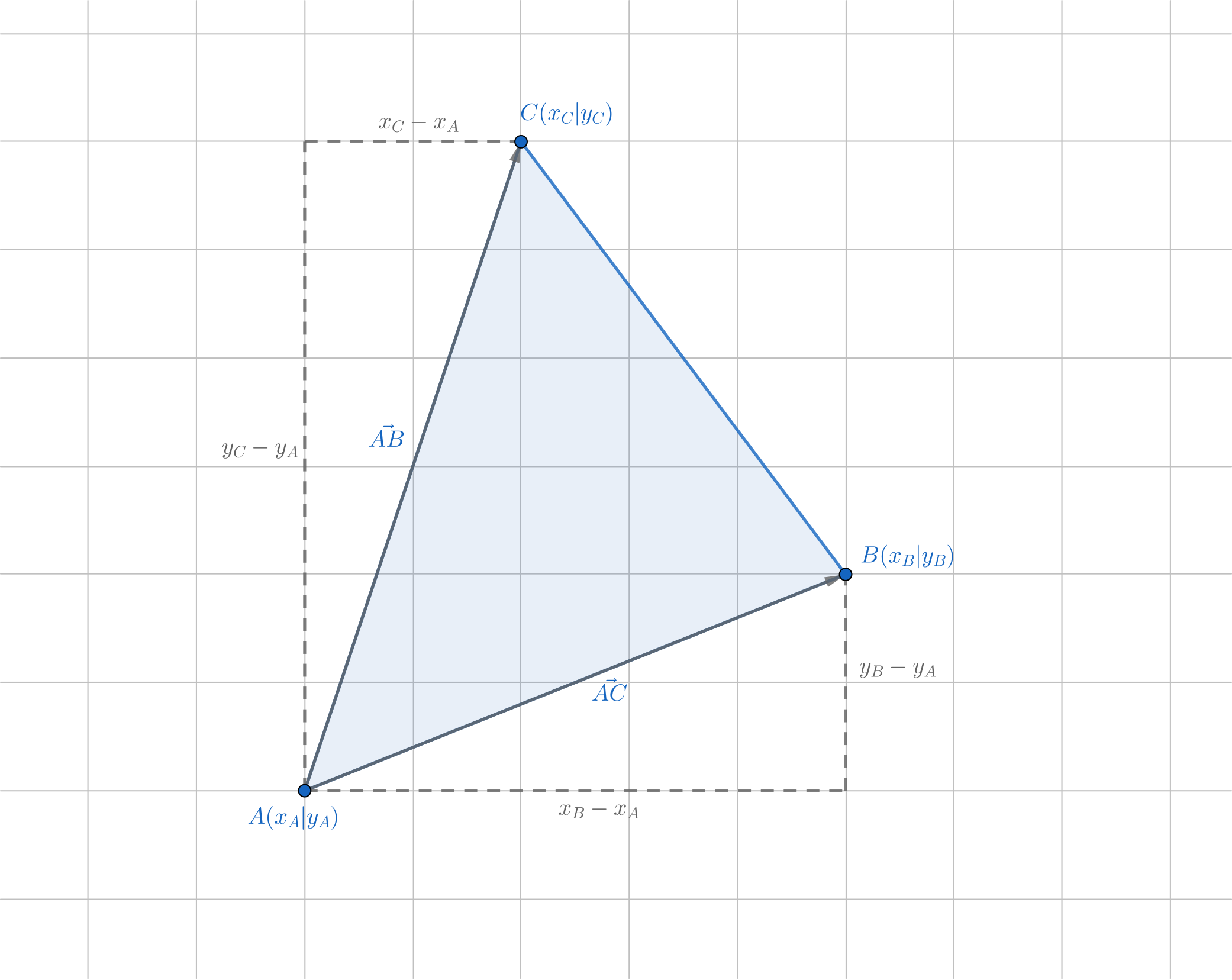The formula most people would be thinking of if they are asked to compute the area of a triangle would probably be $A = \frac{1}{2}bh$. While this formula is indeed very useful, there are situations where calculating the base and the height of a triangle is non-trivial. It would be quite convenient to have a formula that would compute the area of a triangle purely based on coordinates, wouldn’t it? Of course, much smarter people have already found such a formula in the 18th century (in fact, they found a formula that allows you to find the area for any polygon, not just triangles): $A = {\frac {1}{2}}{\big |}(x_{A}-x_{C})(y_{B}-y_{A})-(x_{A}-x_{B})(y_{C}-y_{A}){\big |}$But why does this formula work? To explain this, we’ll build on another way to compute the area, namely using vectors: $A = \frac{1}{2}|\vec{AB} \times \vec{AC} |$. (This formula uses a nice property of the cross product. You can find explanations for it all over the internet.) $\vec{AB} = \begin{pmatrix} x_B - x_ A \\ y_B - y_A \end{pmatrix}$ , and similarly $\vec{AC} = \begin{pmatrix} x_C - x_A \\ y_C - y_A \end{pmatrix}$. Now, we can apply the cross product. As the cross product only works in three dimensions, we’ll add a z-coordinate and set it to 0:

$$\begin{pmatrix} x_B - x_A \\ y_B - y_A \\ 0 \end{pmatrix} \times \begin{pmatrix} x_C - x_A \\ y_C - y_A \\ 0 \end{pmatrix} = \begin{pmatrix} 0 \\ 0 \\ (x_B - x_A)(y_C - y_A) - (x_C - x_A)(y_B - y_A) \end{pmatrix}$$

The first two components are zero and can therefore be ignored. So, let’s put the z-component back into the original formula: $A = \frac{1}{2} \vert (x_B - x_A)(y_C - y_A) - (x_C - x_A)(y_B - y_A)\ \vert$.

What remains now is already very similar to the shoelace formula for triangles - only a little bit of transformation is needed now:

$$A = \frac{1}{2} | \textcolor{red}{-} (\textcolor{red}{-} x_B \textcolor{red}{+} x_A)(y_C - y_A) \textcolor{red}{+} (\textcolor{red}{-} x_C \textcolor{red}{+} x_A)(y_B - y_A)| \newline = {\frac {1}{2}}{\big |}(x_{A}-x_{C})(y_{B}-y_{A})-(x_{A}-x_{B})(y_{C}-y_{A}){\big |}$$

We negate the content of the first parentheses of both terms but also add a minus in front - this means that we have not changed the outcome, but only the notation. Now, our term is identical to the shoelace formula!

This formula can be super useful, especially for optimization problems where two out of three coordinates are given.